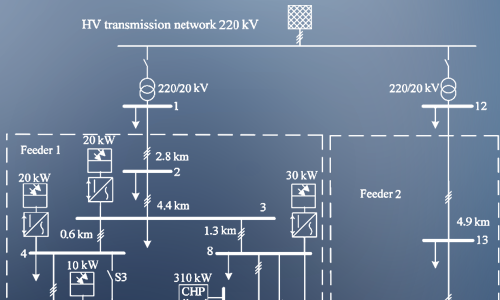To get started with pandapower, just

1. Install pandapower through pip:

pip install pandapower

2. Create a simple network

import pandapower as pp
net = pp.create_empty_network()
b1 = pp.create_bus(net, vn_kv=20.)
b2 = pp.create_bus(net, vn_kv=20.)
pp.create_line(net, from_bus=b1, to_bus=b2, length_km=2.5, std_type="NAYY 4x50 SE")
pp.create_ext_grid(net, bus=b1)

3. Run a power flow:

pp.runpp(net)

4. And check the results:

print(net.res_bus.vm_pu)

But of course pandapower can do much more than that - find out what on this page!Electric Modeling

Includes thoroughly validated equivalent circuit models for lines, transformers, switches and more.Power System Analysis

Supports power flow, optimal power flow, state estimation, short-circuit calculation and topological graph searches.Free and Open

Published under a BSD License and therefore free to use, modify and share however you want.